Courses
Courses for Kids
Free study material
Free LIVE classes
More

# Basic Shapes Around Us## An Insight into Basic shapes - The Building Blocks of Geometry

A pizza is the most ‘shape-filled’ object you’ll ever come across. Coming in a square box, the pizza is a circle itself. Hold up, the best part is yet to come, the slices are triangular!

Let’s just say you’ll realise the epitome of shapes that pizzas are hereafter. Let’s jump right in to understand the basic shapes and their properties.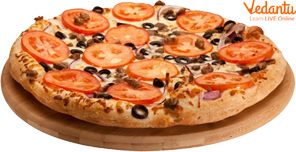Pizza

## Plane Figures and Their Features

Any shape that lies flat on one plane can be called a plane figure. Our good old squares, rectangles, triangles, and circles all come under plane figures.

These shapes have sides, corners, and angles.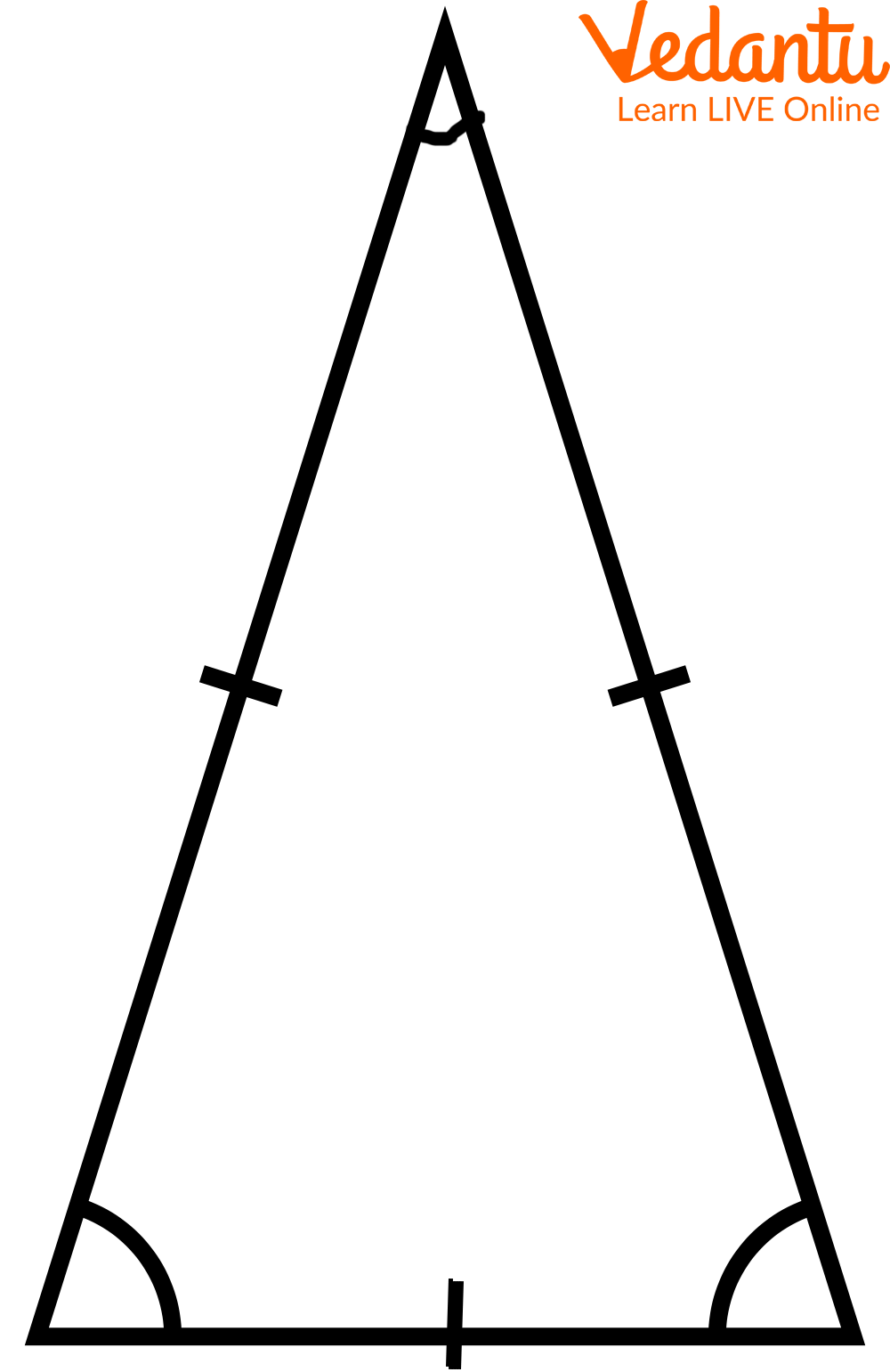Sides and Corners of a Triangle

### Square

The basic shape we’re starting with is that of a square.

A square has the following features:

• 4 equal sides

• 4 corners are placed perpendicularly

• 4 right angles between the sides (remember the L)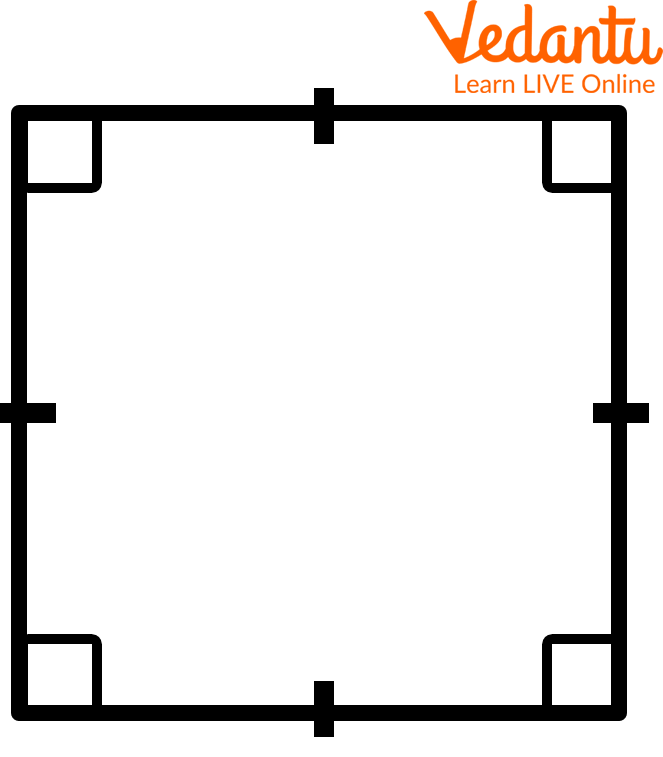Sides, Angles, and Diagonals of a Square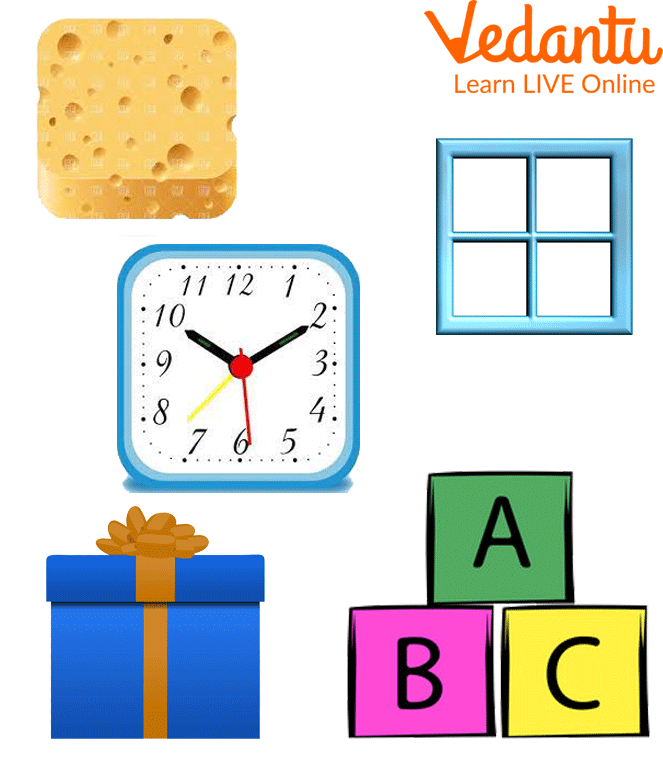Square-shaped Object Around Us

### Rectangle

A rectangle has the following features:

• 4 sides

• Opposite sides are equal to each other

• 4 corners are placed perpendicularly

• 4 right angles are placed between the sides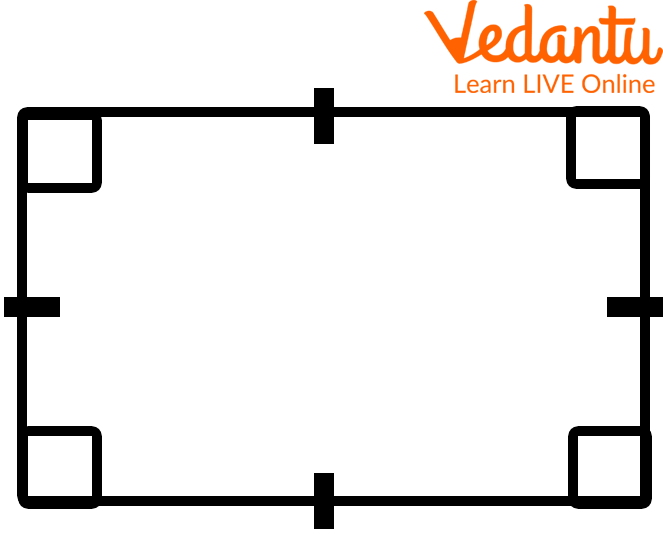Sides and Angles of a Rectangle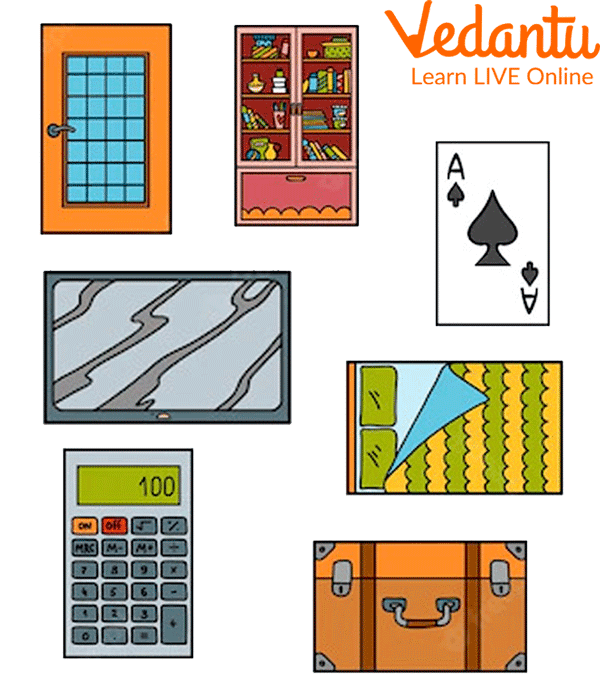Rectangle-shaped Objects Around us

### Triangle

The name says it all for a triangle.

• 3 sides (base and opposite sides)

• 3 corners or vertices

• Diagonals are not possible in a triangle.

Triangles can be further classified as illustrated below.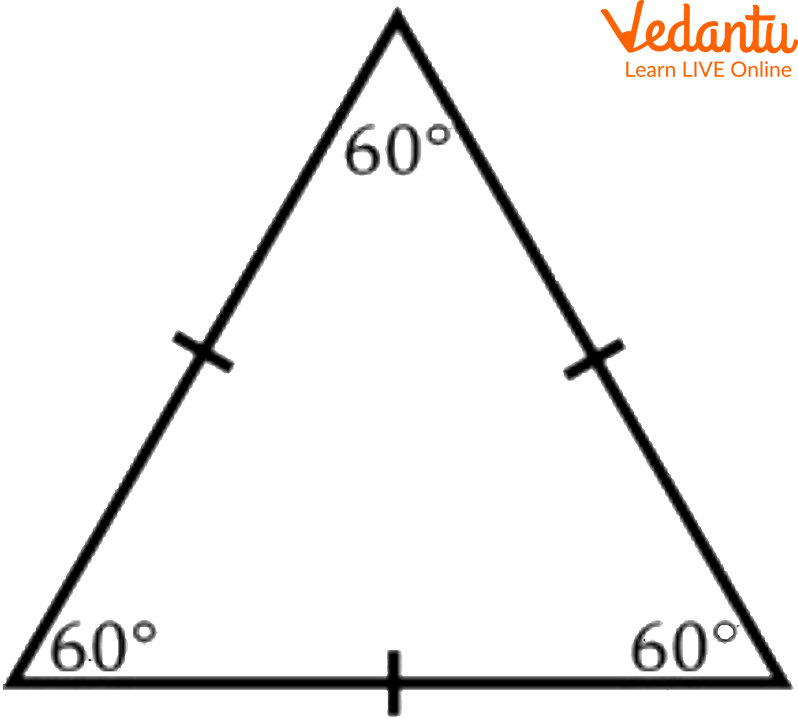Triangle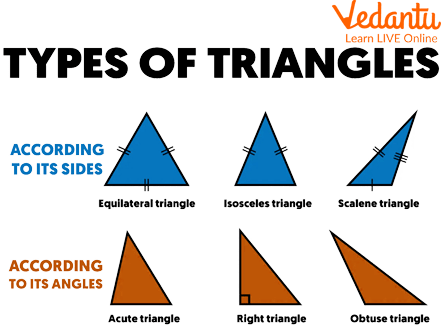Types of Triangle

### Circle

The circle is the hero of the show! No corners, no sides, no diagonals to deal with.

Features of the circle are as follows:

• It is made up of curved lines with an origin

• A diameter divides the circle into 2 equal halves ( it is the longest line that can be fit into it)

• Half of a diameter is called the radius.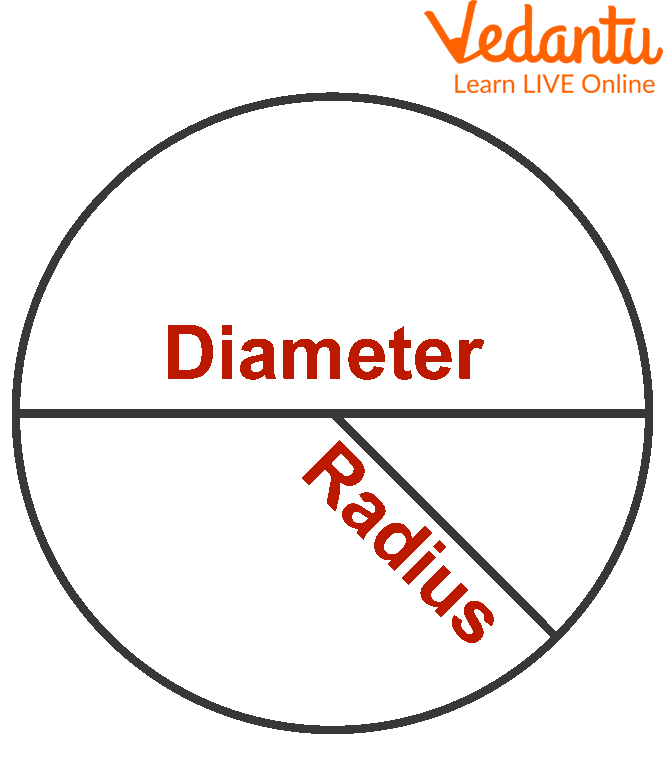Parts of a Circle

Here O is the origin of the circle, line D is the diameter and R is the radius.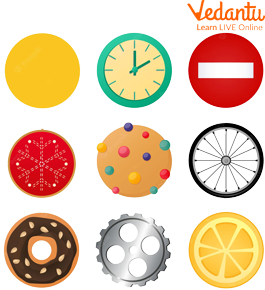Circle-Shaped Objects Around us

## Special Plane Figures

There are several other specialised plane figures that are one way or the other related to squares and rectangles. These are collectively known as quadrilaterals as they have 4 sides (quad meaning 4). Parallelogram, Trapezoid, Rhombus, and Kite fall under this category.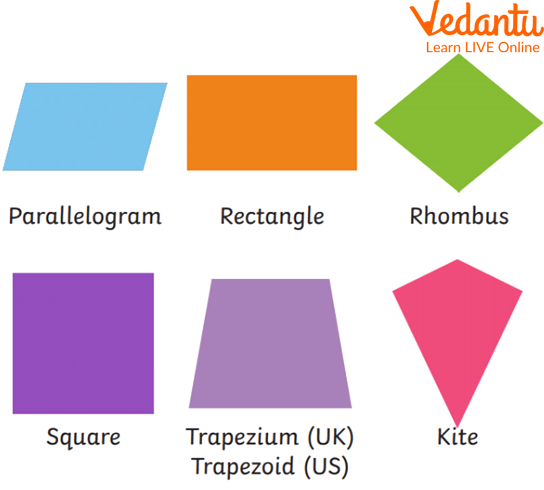## Solid Figures and Their Features

Any shape that occupies space can be called a solid figure. Cubes, cuboids, prisms, cones, spheres, and cylinders fall under this category. These shapes have faces, edges, and vertices which give them their unique features. It is easier to remember solid figures as plane figures put together in all the possible dimensions. So, solid figures also have sides, corners, and angles.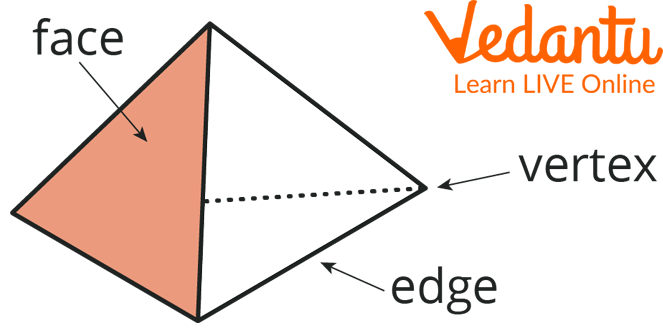Parts of a Solid Shape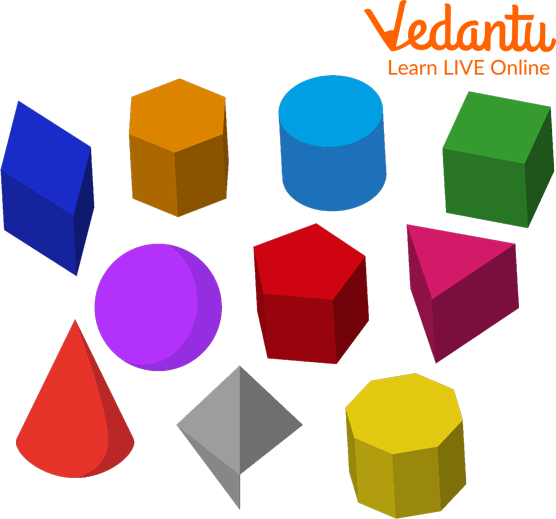Types of Solid Shapes

### Cube

Cubes are made up of squares but in 3 dimensions.

• All faces and sides of a cube are equal.

• The edges are equal.

• There are 6 faces, 12 edges, and 8 vertices in a cube.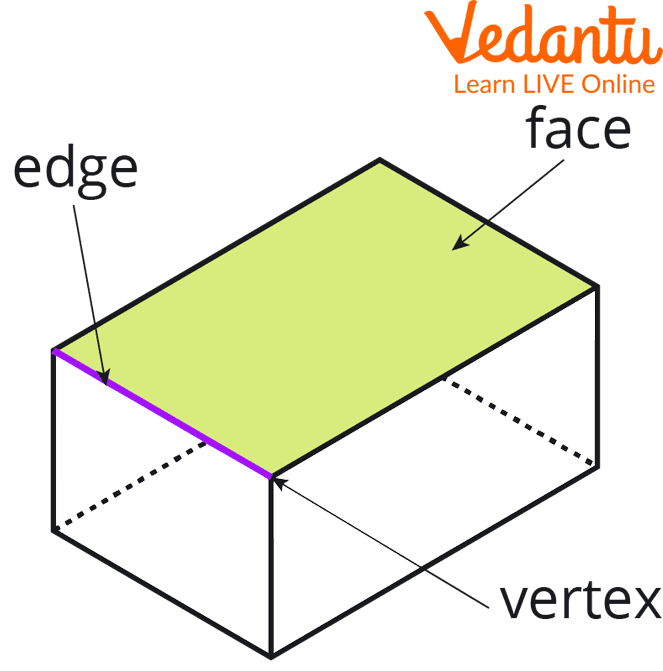Faces, Edges, and Vertices of a CubeCube-Shaped Objects Around us

### Cuboid

Slightly modified cubes give us cuboids.

• The opposite faces of a cuboid are equal.

• Opposite edges are equal.

• There are 6 faces, 12 edges, and 8 vertices in a cuboid.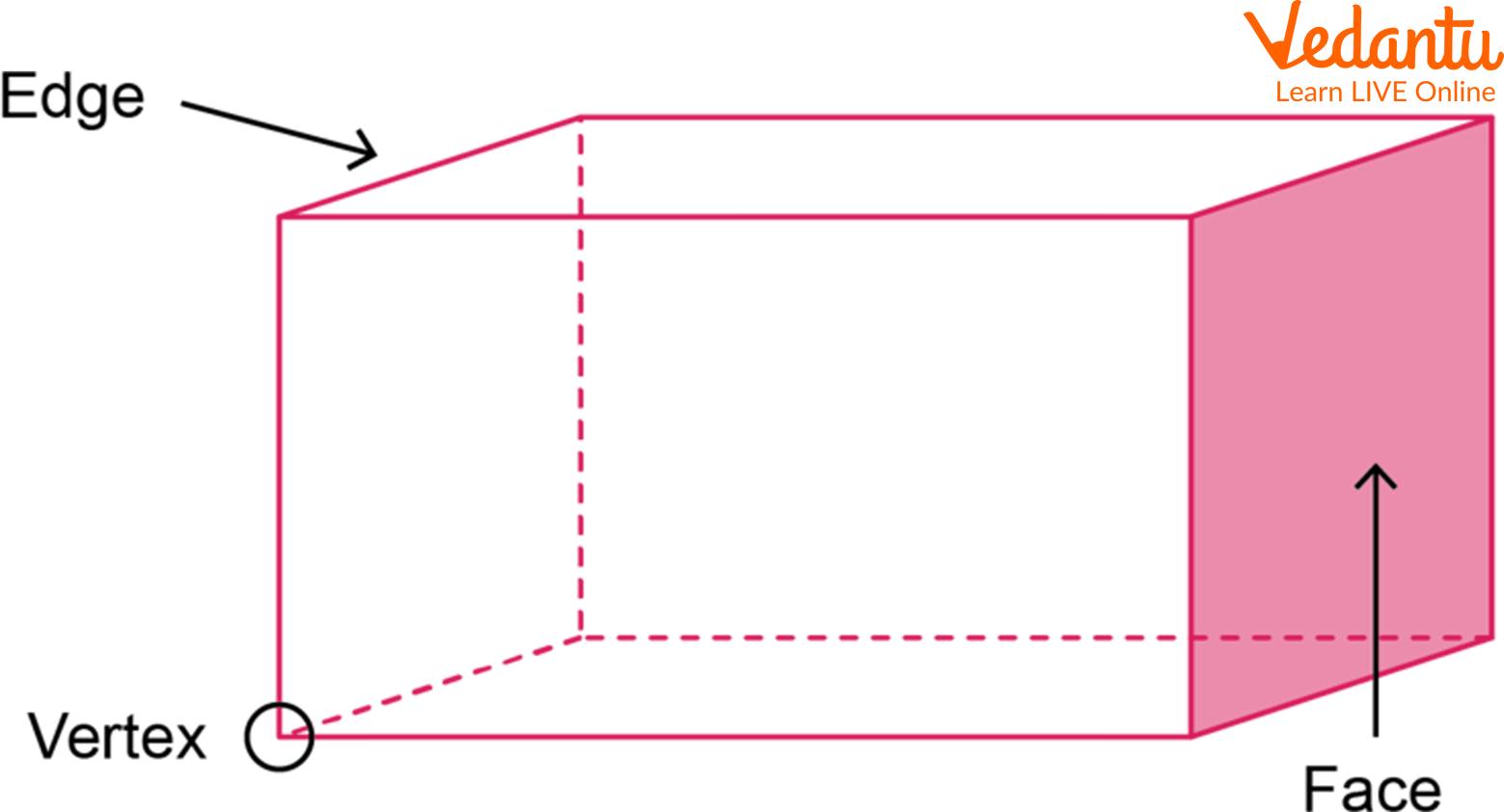Faces, Edges, and Vertices of a Cuboid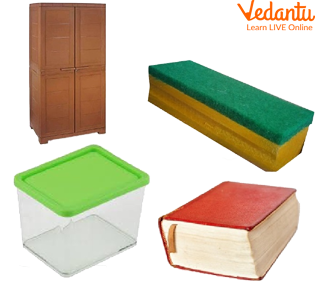Cuboid-shaped Objects

### Cone

Birthday parties are all about cones! Ice cream cones, birthday caps, and whatnot. This peculiar solid shape has the following properties

• It has one curved surface and one flat surface.

• It has one vertex and one curved edge.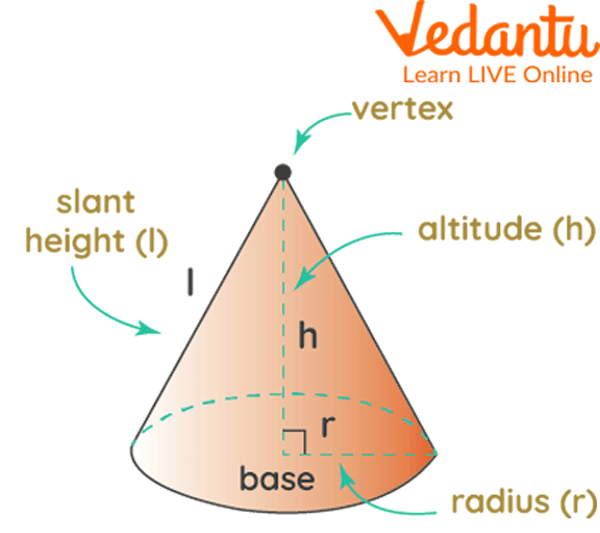Parts of a Cone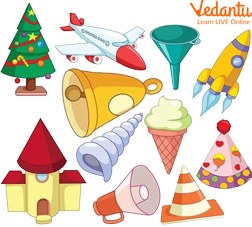Cone-Shaped Objects

### Cylinder

The cylinder is a huge stack of circles placed on top of the other. The properties of a cylinder are as follows:

• Three surfaces

• It has no vertex

• 2 curved edges are present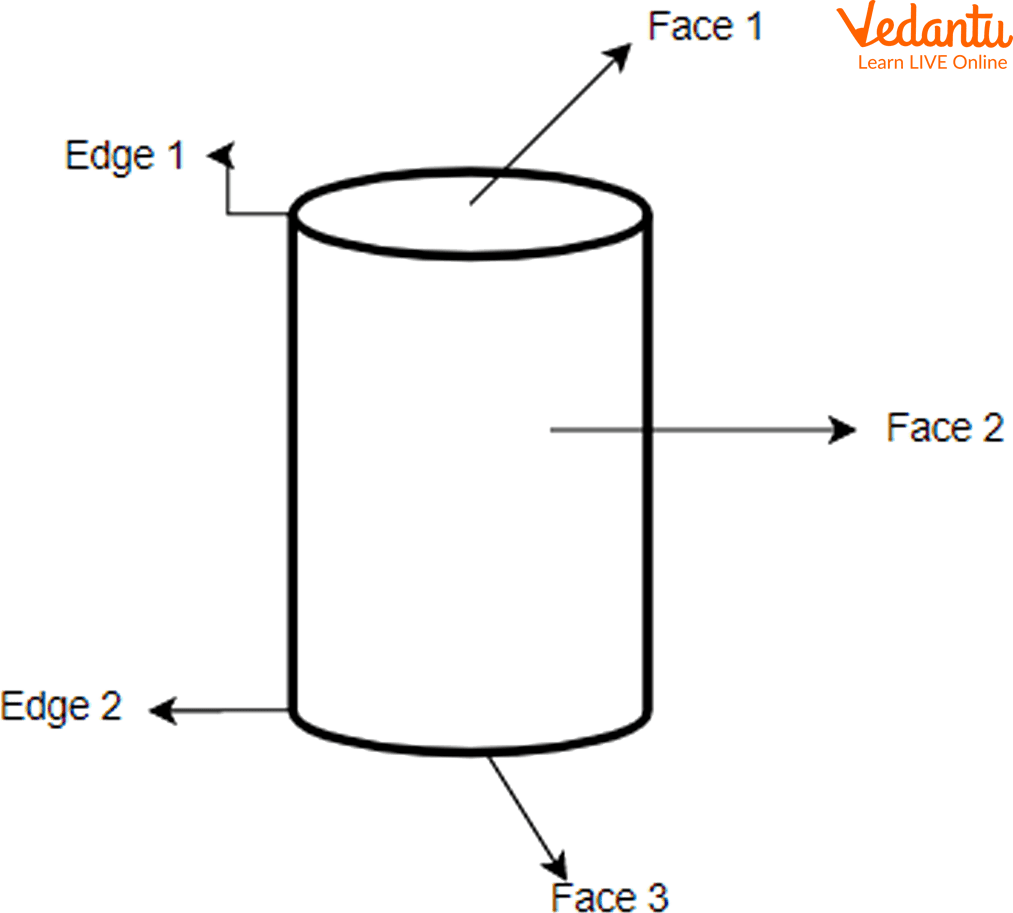Parts of a Cylinder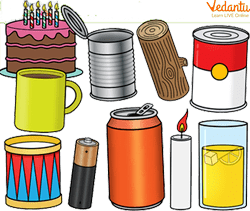Cylinder-shaped Objects

### Sphere

A ball is the best example of a sphere. Properties of the sphere are as follows:

• It has no edges or vertices.

• It has one curved surface area.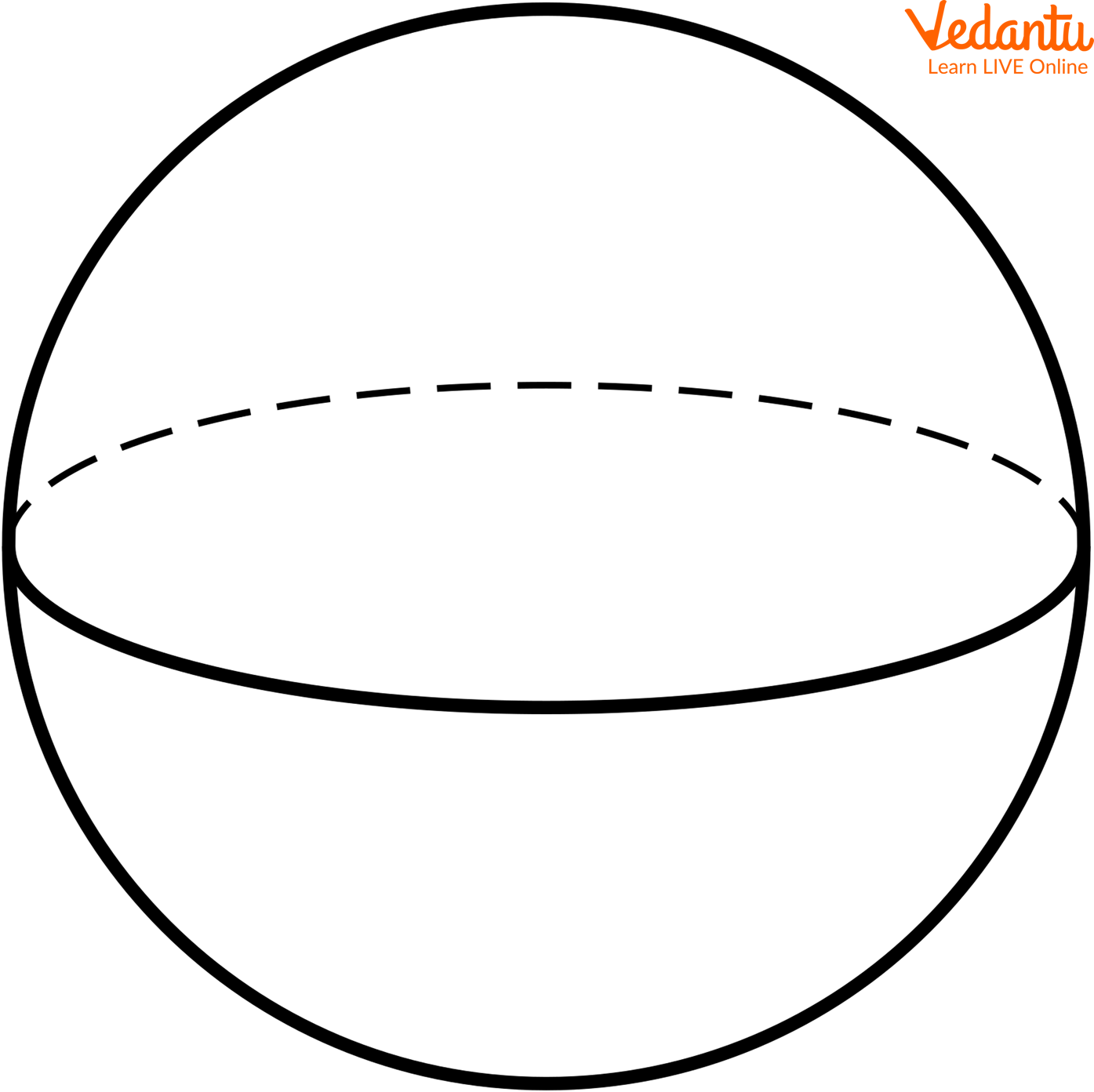Sphere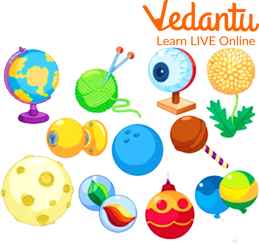Spherical Objects Around Us

## Practice Questions

Q1. ___________________ is a basic shape in which no diagonals are possible (Ans: Triangle)

Q2. _____________ sides are equal in a rectangle (Ans: opposite)

Q3. The longest line possible in a circle dividing the circle into 2 equal halves is called _______________ (Ans: diameter)

## Summary

Basic shapes mainly are composed of plane figures and solid figures. Plane figures, also known as 2-dimensional shapes, lie on a single plane having length and breadth. Examples of plane figures include squares, rectangles, triangles, circles, and quadrilaterals.

Solid shapes include cubes, cuboids, cones, cylinders, and spheres having length, breadth, and height. These are also known as 3-dimensional objects as they occupy space. In common, all the basic shapes have faces, edges, vertices, corners, and angles and are of different types.

Last updated date: 26th Sep 2023
Total views: 124.2k
Views today: 1.24k

## FAQs on Basic Shapes Around Us

1. Is a square a rectangle?

Yes, the square is a special type of rectangle as the sides of a square are all equal. Square has all equal sides and its opposite sides are also equal to each other.

Thus, a square is also a rectangle. However, the opposite isn’t true. A rectangle cannot be square. This is because only the opposite sides of a rectangle are equal to each other, and not all sides of a rectangle are equal to one another. So a square is a special type of rectangle but a rectangle is not a square.

2. How can triangles be further classified based on their sides and angles?

Triangles are classified further based on the length of sides and angles as

(i) Equilateral triangle (all three sides are equal)

(ii) Isosceles triangle (2 adjacent sides are equal)

(iii) Scalene triangle (no two sides are equal)

(iv Acute angled triangle (One angle of the triangle is less than 90 degrees)

(v) Obtuse angled triangle (One angle of the triangle is greater than 90 degrees)

(vi) Right-angled triangle (One angle of the triangle is 90 degrees)